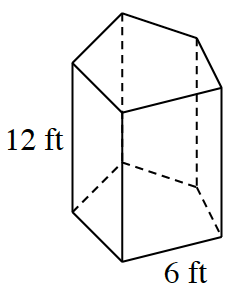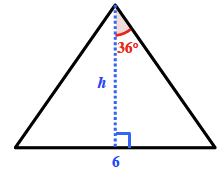### Home > CCG > Chapter 10 > Lesson 10.1.4 > Problem10-46

10-46.Calculate the total surface area and volume of the prism at right. Assume that the base is a regular pentagon.

Find the central angle of the pentagon.

$\frac{360°}{5}=72°$

The area of the triangle will help you find the area of the pentagon.$\text{tan}\left(36°\right)=\frac{3}{h}$$h=\frac{3}{\text{tan}\left(36°\right)}$, $h \approx 4.1291 \; \text{ft}$

$\text{Area of the triangle} \approx \left(0.5\right) \left(4.1291\right) \left(6\right) \approx 12.39 \; \text{ft}^2$
$\text{Area of pentagon} \approx \left(5\right) \left(12.39\right) \approx 61.9 \; \text{ft}^2$

Find the area of each of the sides, and sum them to find the surface area.
$\left(12\right)\left(6\right)\left(5\right)=360$
$360 + 2 \left(61.9\right) \approx 483.8 \; \text{ft}^2$

Multiply the area of the pentagon by the height to find the volume.
$\left(61.9\right)\left(12\right)\approx743.3\text{ ft}^3$

$\text{Volume} \approx 743.3 \; \text{ ft}^3$
$\text{Surface area} \approx 483.3 \; \text{ft}^2$